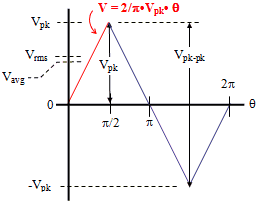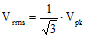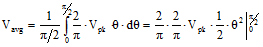# Triangle Wave Voltages - Vpk, Vpk-pk, Vavg, Vrms

When plotted as voltage (V) as a function of phase (θ), a triangle wave looks similar to the figure to the right. The waveform repeats every 2π radians (360°), and is symmetrical about the voltage axis (when no DC offset is present). Voltage and current exhibiting cyclic behavior is referred to as alternating; i.e., alternating current (AC). One full cycle is shown here. The basic equation for a triangle wave is as follows:for 0 ≤ θ < π/2

There are a number of ways in which the amplitude of a triangle wave is referenced, usually as peak voltage (Vpk or Vp), peak-to-peak voltage (Vpp or Vp-p or Vpkpk or Vpk-pk), average voltage (Vav or Vavg), and root-mean-square voltage (Vrms). Peak voltage and peak-to-peak voltage are apparent by looking at the above plot. Root-mean-square and average voltage are not so apparent.Also see Sinewave Voltages and Square Wave Voltages pages.

Root-Mean-Square Voltage (Vrms)

As the name implies, Vrms is calculated by taking the square root of the mean average of the square of the voltage in an appropriately chosen interval. In the case of symmetrical waveforms like the triangle wave, a quarter cycle faithfully represents all four quarter cycles of the waveform. Therefore, it is acceptable to choose the first quarter cycle, which goes from 0 radians (0°) through π/2 radians (90°).

Vrms is the value indicated by the vast majority of AC voltmeters. It is the value that, when applied across a resistance, produces that same amount of heat that a direct current (DC) voltage of the same magnitude would produce. For example, 1 V applied across a 1 Ω resistor produces 1 W of heat. A 1 Vrms triangle wave applied across a 1 Ω resistor also produces 1 W of heat. That 1 Vrms triangle wave has a peak voltage of √3 V (≈1.732 V), and a peak-to-peak voltage of 2√3 V  (≈3.464 V).

Since finding a full derivation of the formulas for root-mean-square (Vrms) voltage is difficult, it is done here for you.So,≈ 0.577 Vpk= 0.57735026918962576450914878050196= 1.7320508075688772935274463415059

Average Voltage (Vavg)

As the name implies, Vavg is calculated by taking the average of the voltage in an appropriately chosen interval. In the case of symmetrical waveforms like the triangle wave, a quarter cycle faithfully represents all four quarter cycles of the waveform. Therefore, it is acceptable to choose the first quarter cycle, which goes from 0 radians (0°) through π/2 radians (90°).

As with the Vrms formula, a full derivation for the Vavg formula is given here as well.So,≈ 0.5 VpkAbout RF CafeCopyright: 1996 - 2024Webmaster:    Kirt Blattenberger,    BSEE - KB3UON RF Cafe began life in 1996 as "RF Tools" in an AOL screen name web space totaling 2 MB. Its primary purpose was to provide me with ready access to commonly needed formulas and reference material while performing my work as an RF system and circuit design engineer. The World Wide Web (Internet) was largely an unknown entity at the time and bandwidth was a scarce commodity. Dial-up modems blazed along at 14.4 kbps while typing up your telephone line, and a nice lady's voice announced "You've Got Mail" when a new message arrived... All trademarks, copyrights, patents, and other rights of ownership to images and text used on the RF Cafe website are hereby acknowledged. My Hobby Website:  AirplanesAndRockets.comPlease Support RF Cafe by purchasing my  ridiculously low−priced products, all of which I created. These Are Available for Free